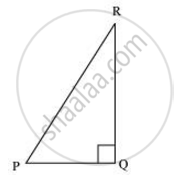Share

# In ΔPQR, right angled at Q, PR + QR = 25 cm and PQ = 5 cm. Determine the values of sin P, cos P and tan P. - CBSE Class 10 - Mathematics

#### Question

In ΔPQR, right angled at Q, PR + QR = 25 cm and PQ = 5 cm. Determine the values of sin P, cos P and tan P.

#### Solution

Given that, PR + QR = 25

PQ = 5

Let PR be x.

Therefore, QR = 25 − xApplying Pythagoras theorem in ΔPQR, we obtain

PR2 = PQ2 + QR2

x2 = (5)2 + (25 − x)2

x2 = 25 + 625 + x2 − 50x

50x = 650

x = 13

Therefore, PR = 13 cm

QR = (25 − 13) cm = 12 cm

sin P = (QR)/(PR)=12/13

cos P = (PQ)/(PR)=5/13

tan P = (QR)/(PQ)=12/5

Is there an error in this question or solution?

#### APPEARS IN

NCERT Solution for Mathematics Textbook for Class 10 (2019 to Current)
Chapter 8: Introduction to Trigonometry
Ex. 8.10 | Q: 10 | Page no. 181
Solution In ΔPQR, right angled at Q, PR + QR = 25 cm and PQ = 5 cm. Determine the values of sin P, cos P and tan P. Concept: Trigonometric Ratios.
S